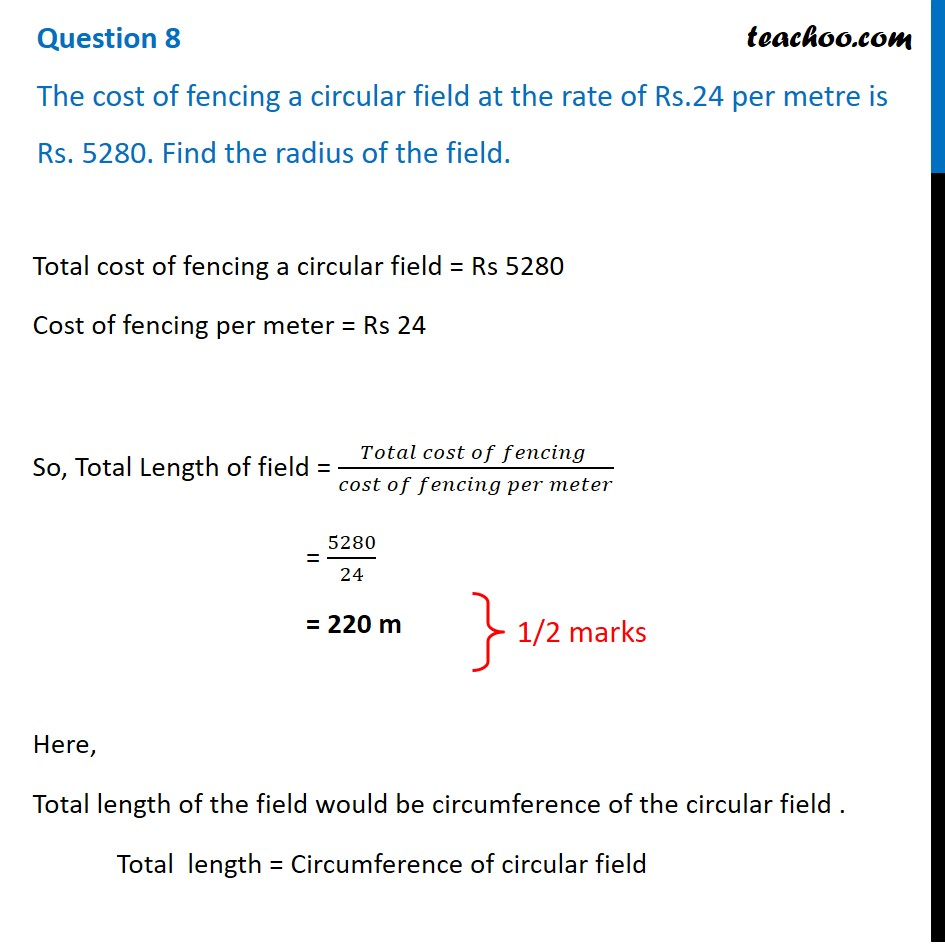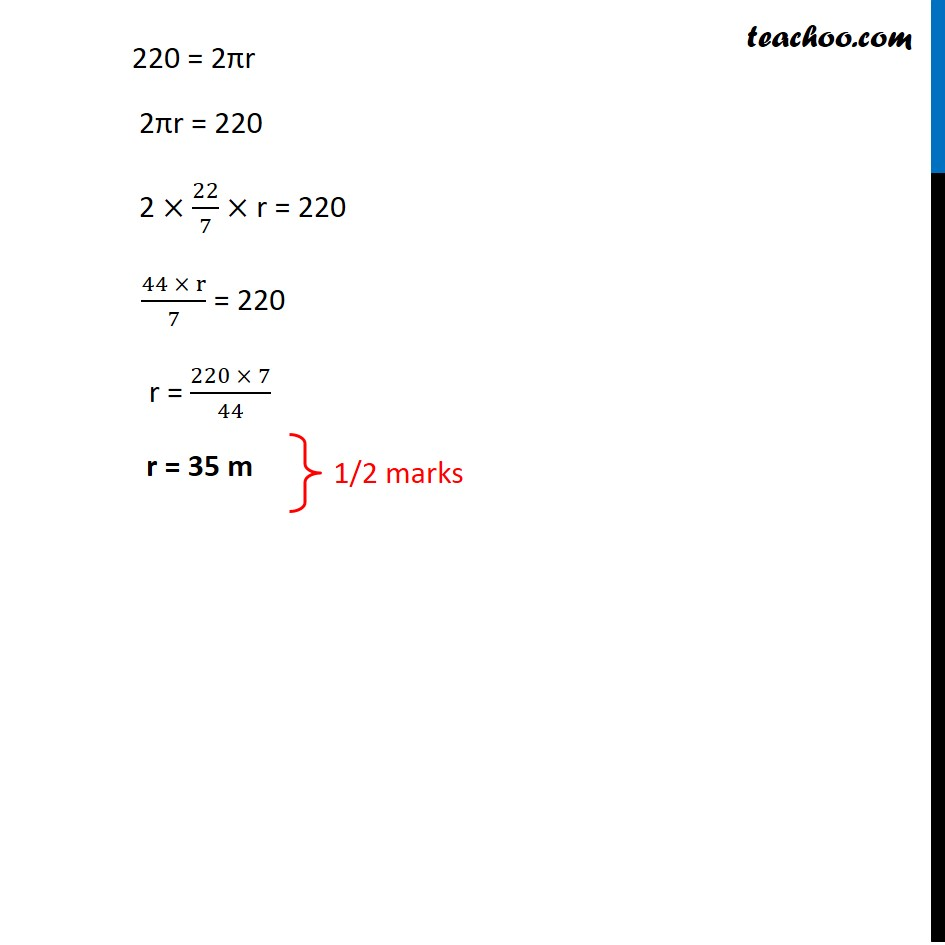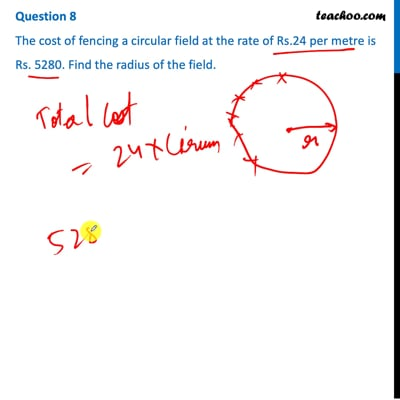CBSE Class 10 Sample Paper for 2021 Boards - Maths Basic

Class 10
Solutions of Sample Papers for Class 10 Boards

## The cost of fencing a circular field at the rate of Rs.24 per metre is Rs. 5280. Find the radius of the field.This video is only available for Teachoo black users

Note : This is similar to Example 1 of NCERT – Chapter 12 Class 10

### Transcript

Question 8 The cost of fencing a circular field at the rate of Rs.24 per metre is Rs. 5280. Find the radius of the field.Total cost of fencing a circular field = Rs 5280 Cost of fencing per meter = Rs 24 So, Total Length of field = (𝑇𝑜𝑡𝑎𝑙 𝑐𝑜𝑠𝑡 𝑜𝑓 𝑓𝑒𝑛𝑐𝑖𝑛𝑔 )/(𝑐𝑜𝑠𝑡 𝑜𝑓 𝑓𝑒𝑛𝑐𝑖𝑛𝑔 𝑝𝑒𝑟 𝑚𝑒𝑡𝑒𝑟) = 5280/24 = 220 m Here, Total length of the field would be circumference of the circular field . Total length = Circumference of circular field 1/2 marks 220 = 2πr 2πr = 220 2 ×22/7× r = 220 (44 × r)/7 = 220 r = (220 × 7)/44 r = 35 m 1/2 marks